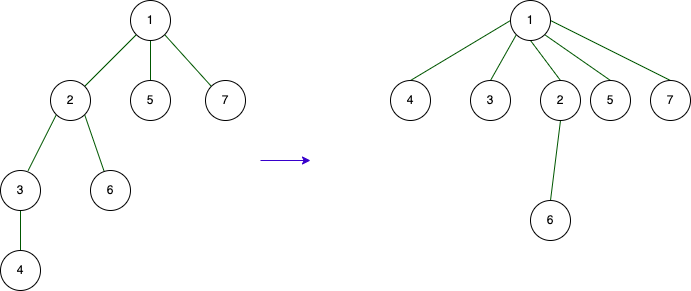About the Disjoint Set Union (Union Find) data structure:

Judging by its name, the data structure consists of three words: union, find, and disjoint. There are three main operations or concepts associated with it.

1. Determining to which part it belongs
2. Union, which involves combining two separate parts.

The structure has two actions. Initially, we start with multiple separate parts and the goal is to connect them easily while being able to determine to which part it belongs.

## Structure#

What structure can be used to accomplish this easily? In other words, let’s assume that vertices within the same area are identical and denoted as R. If a new element is added to that section, we only need to mark that element as R. However, a problem arises when there is an X element in the first part and a Y element in the subsequent part. This can be resolved by taking the minimum of X and Y, where all elements in the smaller section are assigned the value of the larger section. So, our proposed structure is as follows:

1. Find - performing 1 operation.
2. Merge - when two parts are combined, the same operation is applied to the smaller number of elements from the two parts.

But if the two separate parts contain a large number of elements, the process will inevitably take longer. We need a more efficient data structure for improved performance. In such cases, we can employ a tree structure to store the elements. A tree is a type of connected graph that doesn’t contain cycles, as elaborated in my article on graphs. The construction of the tree structure follows these steps:

• Select a vertex as the representative of the separate part, known as the root.
• Each vertex within the section indicates its parent vertex.
• The highest-level parent is either the root (the representative of the separate part)

How can we merge two separate parts using this structure?

• Find the root of vertex X. In doing so, it will traverse its parent.
• Find the root of vertex Y. In doing so, it will traverse its parent.
• When merging, the representative or root vertex of X will become the parent of the representative or root vertex of Y.

In terms of time complexity, the merging process becomes a chain of find operations, with each find operation potentially taking time proportional to the height of the tree. How can we make it faster? During the search process, as we traverse the parent pointers, we can optimize it by updating the immediate parent of each vertex to point directly to the root vertex. In other words, when we perform the root-finding operation, all vertices along that path will immediately point to the root vertex. This reduces the height of the tree and speeds up future find operations.After executing the find from index 4

This image shows what it looks like after finding from vertex 4. Union-find can perform search and union in almost 1 operation, making it highly efficient.

## Example problem#

Assume that we initially have a graph in which no two vertices are connected. In other words, there are a total of N components. Let’s consider an example problem of connecting two vertices, x and y, with a total of Q connections and finding the total number of components after all these connections have been made.

``````#include <bits/stdc++.h>
ing namespace std;

const int N = 200000;

int n
int q;
int s[N];
int comp;
int par[N];

int find(int x) {
if(x == par[x]) {
return x;
}
int root = find( par[x] );
par[x] = root;
return root;
}

int main() {
cin >> n;
comp = n;

for(int i = 1; i <= n; i++) {
par[i] = i;
s[i] = 1;
}

cin >> q;

while(q--) {
int x, y;
cin >> x >> y;

int rootX = find(x);
int rootY = find(y);

if(rootX == rootY) {
// do nothing, cause there are in same component
} else {
comp--;
if(s[rootX] > s[rootY]) {
par[rootY] = rootX;
s[rootX] += s[rootY];
s[rootY] = 0;
} else {
par[rootX] = rootY;
s[rootY] += s[rootX];
s[rootX] = 0;
}
}
cout << comp << endl;
}

return 0;
}
``````